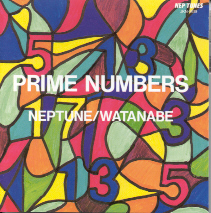# 17

This number is a prime.

Just showing those entries submitted by 'Rivera': (Click here to show all)Prime Numbers CD Image J. Neptune © 199417 is the only odd prime p such that p + sod(p) and p - sod(p) are square numbers (52 and 32, respectively). [Rivera]The smallest automorphic prime, i.e., a prime p such that p is the kth prime and p ends in k. [Russo and Rivera]17 is the minimal prime solution as sum of three distinct triangular integers: 17=1+6+10. [Rivera]